# Action Research in A Smart Way via Smartphones: The New Mode to Meliorate Teachers

London Journal of Research in Computer Science and Technology
Volume | Issue | Compilation
Authored by R. Nageswari , Hovakimyan Anna
Classification: C.2.6
Keywords: neural network, the weight of the neuron, recognition of the stimulus, mathematical expectation, dispersion.
Language: English

Is smartphone an aid to improve the teacher performance of tertiary level? Do the observation practices in group through smartphone applications help in improving oneself and a team of teachers simultaneously? The presentation analyses the impact of using smartphones and instant messaging applications for action research to improve the quality of performance of tertiary level teachers. The paper focuses on the practice of group assessments of teachers for betterment of English learning process in classrooms.

# Statistical Evaluation of the Performance of the Neural Network

## _____________________________________________

1. ### ABSTRACT

In this paper the problem of evaluating the performance of the neural network, based on a study of the probabilistic behavior of the network is considered. Direct propagation network consisted of layer of input nodes, hidden layer and output layer is examined. To evaluate the network performance the mathematical expectation and dispersion of weight at the input of the output layer are considered. For such networks the estimates for some of the statistical characteristics of the neural network in the case of two recognized classes were obtained.

Keywords: neural network, the weight of the neuron, recognition of the stimulus, mathematical expectation, dispersion.

Author α σ: Department of Programming and Infor- mation Technologies Yerevan State University, Yerevan, Armenia.

1. ### INTRODUCTION

Artificial neural networks are often used for a variety of applications. For the successful application of artificial neural networks it is necessary to choose the right network architecture, to pick up its parameters, thresholds elements, activation function, and others [1, 2, 4]. Currently neural computation of different level of implementation, from specialized hardware to the neural network software packages, are becoming more widely used.

They are successfully used to solve a number of tasks such as forecasting of economic and financial indicators, the prediction of complications in patients in the postoperative period, biometric identification based on various characteristics, image processing, and others.

All this is possible for the neural networks because of their ability to learn and to establish of associative connections in the input data. Typically, depending on the task, it is required to resort to different methods to transform input data, allowing a correct judgment about the laws and special features in the data that reflect their quality characteristics .

1. ### PROBLEM DESCRIPTION

Artificial neural network is a mathematical model of biological neuron. It consists of relating to each other neurons. A neural network is exposed to learning by providing it with the input information in the form of numerical sequences. The training set up internal connections between neurons through which the network is endowed ability to recognize unfamiliar images. There are different types of neural networks that differ in both the topology and the learning algorithms.

In the neural network technologies an important role particularly play  the selection of the network architecture and the values of its parameters that affect its efficiency.

A particular interest the study of the probabilistic behavior of the neural networks is presented [3, 4]. The neural network of direct distribution, which consists of a layer of input nodes, hidden layer and output layer is investigated. Neurons have a one-way communication, do not contain links between elements within the layer and backward linkages between the layers. The neurons of the input layer are connected to the hidden layer neurons with excitatory and inhibitory connections randomly. The outputs of all neurons in the hidden layer neurons are connected to the output layer. Neurons in each layer are referred to as the input, hidden and output elements, respectively [1, 2, 4]. Neurons have unidirectional links and do not contain links between elements within a layer and feedbacks between the layers.

Signal (causative agent, incentive, stimulus) supplied to the input layer of neural network corresponds to external stimuli and is modeled by the vector whose coordinates take the values 0 and 1, depending on whether or not the corresponding neuron of the input layer is excited. The output signal from each neuron of the input layer is set to 1 if the neuron is excited, and 0 otherwise. The output signal generated by the input layer, is transferred to the hidden layer. Each neuron of the hidden layer implements a threshold function η as it inputs :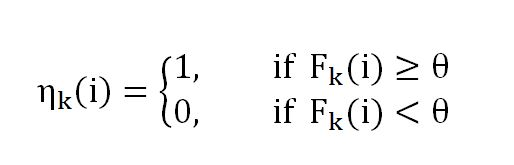where σn and σm are numbers of excited and inhibited bonds from excitatory and m inhibitory connections respectively, θ is threshold of hidden element (integer number). Excitatory and inhibitory connections between the input and hidden layers are assumed to be randomly and uniformly distributed. The outputs of all elements of the hidden layer are passed as an input to the output layer that implements the function R:where ηk is the activity the k-th hidden element, vk is the weight of the k-th hidden element, NA is the number of elements in the hidden layer, and θR is the threshold of the output element.

The structure of the neural network allows to correct errors during incorrect responses by means of gradual complication of decision rules. This is done by changing the weight vectors.

Let us determine the weight of the hidden element after training.

The activity ηk(i) of k-th hidden element when the stimulus ξi is submitted to the network is determined by the formulawhere Fk(i) is the value of signal of the k-th hidden element, and θ is the threshold of hidden element.

At the stage of neural network training, a training sequence of stimuli is presented to network. For each stimulus a hidden element is tested for activity, and all the active elements of the hidden layer are encouraged with values δi (i = 1, 2) for the i-th class of stimuli.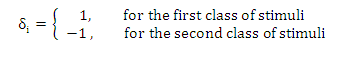If each class has, respectively, l1 and l2 representatives, then after training via the sequence  of two classes activators of the length L = l1+ l2 ,  in the k-th hidden item  the weight  Vk is accumulated: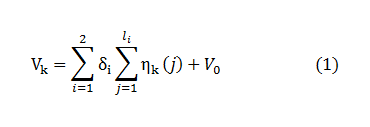where V0- is a initial weight of k –th hidden element.

When at the input to a network is  the stimulus  ξt , so  the input of the output layer is fed weight (k=1, …NA)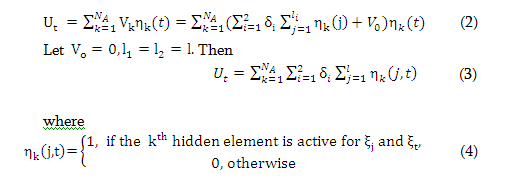The belonging of  pathogen ξt  to one of two classes is determined by comparing of the weight Ut with a threshold output element R. When UtR  so we have first class. When U<θR  - second class. When Ut= θR - refusal of recognition.

For the given network, a predetermined training sequence and a predetermined reference stimulus ξt, the weight Ut has a certain value. However, for the class of neural networks the Ut  is a random variable. To determine the probability, that the network selected from a class, correctly classifies stimulus ξx, is required to calculate the probability characteristics of the random variable of weight Ux, supplied to the input of the output layer.

According to the modified Chebyshev inequality , for any random variable z with mathematical expectation Mz=µ  with any dispersion Dz= σ2 we have following relations:

P(z>0)>= 1- 1/( µ22))=1- σ22, when µ>0                (5)

P(z<0)>= 1- 1/( µ22))=1- σ22, when µ<0                (6)

where P – is the probability of the corresponding event, σ- average quadratic deviation. Equations (5,6) can be used to estimate the probability of a correct response of network on ξx with θR=0 in the case of two recognized classes.

From the (5, 6) it follows that the probability of correct recognition  increases, if  attitude σ22   tends to zero.

With appropriate probabilistic characteristics of the network, we can estimate the probability of a correct  response  of  network  on ξx. If the relation

σ2(Ux)/ µ2(Ux) can be made arbitrarily small, then for a selected network with θR=0 the probability that a stimulus ξx  is classified correctly  seeks to 1.

Let NA is number of hidden elements of the network, L=li+lj, li(lj)-is the length of the training sequence of i-th(j- th) class, δij)- is the increment  of weight of hidden element when the stimulus from the i-th(j -th) class  is presented. Pi is probability of excitation of hidden element when a stimulus from the i –th class is presented, Pij- is probability of excitation of hidden element when  incentives from the both i-th and j-th classes are presented. To find the expectation of the weight  at the input of the output layer the following theorem is proved .`

Theorem. Let the a set of pathogens Ω={ ξ} , Ω=Ω1 U Ω2, Ω1 ∩ Ω2=Ø, and training sequence ξ1,ξ2, …ξL are given. Then the expectation of the weight input in the output layer when the stimulus is from the i –th class is equal to

µi = NA (δiPi li + δjPi jlj);   i,j = 1,2 i≠j             (7)

Having µi, let’s estimate dispersion of random variable Ux with appearance of the pathogen ξx at the entrance of the network.`

For j = 1,..L, and r= 1,..L we receive:

σ2(Ux)=NAL2∑∑νjνrδjδr(Pjrx-Pjx*Prx)       (8)

where Pjx- is probability of excitation of hidden element when displaying incentives ξjx .

Prx- is excitation probability of hidden element when displaying incentives ξr , ξx,

Pjrx- excitation probability of hidden element when displaying incentives ξj , ξr , ξx.

Since the ratio σ2(Ux)/μ2(Ux) is not depend on the length of the training sequence, so any number of repetitions of the same training sequence does not change the characteristics of the system.

1. ### RESULTS

Let’s consider the relation σ2(Ux)/μ2(Ux) for the control  stimulus ξx  in cases, if  ξx € Ω1 and ξx € Ω2.

Assessing the probability Pjrx,Pjx,Prx the expressions for the dispersion will be recieved.

Selected the following cases:

First case: assume that the control stimulus ξx € Ω1. For the stimuli ξj and ξr in this case we obtain the following probabilities:

a) if ξj € Ω1 and ξr € Ω1, so Pjrx= Pjx = Prx = P1;

b) if ξj € Ω1 and ξr € Ω2, so Pjrx= P12 , Pjx = P1 ,Prx = P12;

c) if ξj € Ω2 and ξr € Ω1, so Pjrx= P12 , Pjx = P12 , Prx = P1;

d) if ξj € Ω2 and ξr € Ω2, so Pjrx= P12 , Pjx = P12 ,Prx = P12.

Let’s calculate follow relationship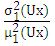for these case (a,b,c,d):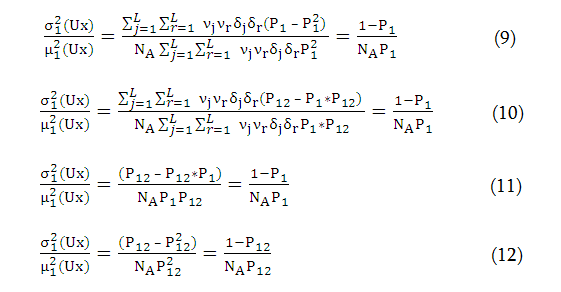The probability of detection for the first class is increased by the maximum value of P1- P12, called the characteristic function of the perceptron (CFP) . Obviously, the function CFP seeks to a maximum value at P1→1.

In assessing the value of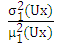again see that P1→1 when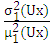→0 (in most of the discussed cases). For the first class of stimuli the condition→0  is received at which execution CFP →max, i.e. the probability of correct recognition for the first class stimulus increases.

Second case: assume that the control stimulus ξx € Ω2.

For the stimulus ξj and ξr in discussed case the following probability will be obtained:

a).  if ξj € Ω2 and ξr € Ω2, so Pjrx = Pjx = Prx = P2;

b).  if ξj € Ω1 and ξr € Ω2, so Pjrx = P12 ,Pjx = P12 ,Prx = P2;

c).  if ξj € Ω2 and ξr € Ω1, so Pjrx = P12 ,Pjx = P2 ,Prx = P12;

d).  if ξj € Ω1 and ξr € Ω1, so Pjrx = P12 ,Pjx = P12 ,Prx = P12.

Similarly, calculating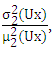, for the second class will be received the condition for correct recognition: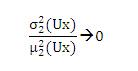Therefore we received new conditions to  increase of the right recognition for the stimulus of the i-th class: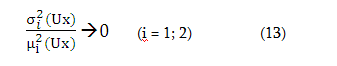### V.  CONCLUSIONS

The resulting estimates for certain statistical characteristics of a neural network in the case of two recognized classes have shown efficiency in the training of neural networks. Moreover, a new condition for improving accuracy of recognition for the stimulus i-th class is received.

### REFERENCES

1. Panteleev S.V. Development, research the use of neural network algorithms, M: 2001, 496 p. (in Russian).
2. Barsky A.B. Neural networks: the recognition, management, decision-making. M .: Finance and Statistics, 2004, 176 p. (in Russian).
3. Sargsyan S.G. Determination of the probability characteristics of adaptive recognition system, Trans. of Intern. Conf. Adaptable software, Kishinev, 1990.pp. 46-51. (in Russian).
4. Ivakhnenko A.G. Perceptron pattern recognition system, Naukova Dumka, Kiev, 1975.p. 426. (in Russian).
5. Hovakimyan A.,Sargsyan S.,Nazaryan A. Self-Organizing Map Application for Iris Recognition . Journal of Commun & Comput. Eng.ISSN 2090-623, www.m-sciences.com, Volume 3,Issue 2. 2013. PP.10-13.
6. Feller V., Introduction to probability theory and its applications, M., Mir, 1984, (in Russian).#### For Authors

/symposiums/webinars, networking opportunities, and privileged benefits.
Authors may submit research manuscript or paper without being an existing member of LJP. Once a non-member author submits a research paper he/she becomes a part of "Provisional Author Membership".

Know more#### For Institutions

Society flourish when two institutions come together." Organizations, research institutes, and universities can join LJP Subscription membership or privileged "Fellow Membership" membership facilitating researchers to publish their work with us, become peer reviewers and join us on Advisory Board.

Know more#### For Subscribers

Subscribe to distinguished STM (scientific, technical, and medical) publisher. Subscription membership is available for individuals universities and institutions (print & online). Subscribers can access journals from our libraries, published in different formats like Printed Hardcopy, Interactive PDFs, EPUBs, eBooks, indexable documents and the author managed dynamic live web page articles, LaTeX, PDFs etc.

Know more